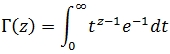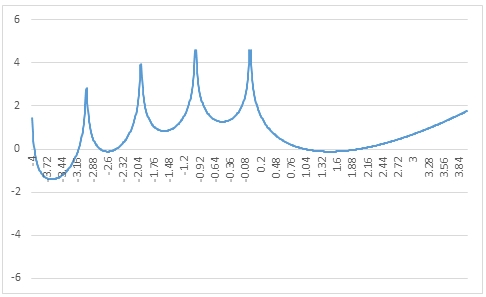# SQL Server GAMMALN function

GAMMALN
Updated: 30 May 2017

Use the SQL Server scalar function GAMMALN to calculate the natural logarithm of Γ(z). The GAMMA function can be defined as a definite integral for R[z] > 0.Syntax
SELECT [wct].[GAMMALN] (
<@Z, float,>)
Arguments
 Input Name Definition @z The value of interest
Return Type
float
Remarks
Examples
Example #1
SELECT wct.GAMMALN(2.5) as Gammaln

This produces the following result.

Example #2
SELECT wct.GAMMA(-3.75) as Gammaln

This produces the following result.

Example #3

The following SQL can be run using the SeriesFloat function to create GAMMALN values from -4 to 4 which can then be pasted into Excel and graphed.

SELECT
z,
wct.GAMMALN(z) as Gammaln
FROM (
SELECT
SeriesValue as z
FROM
wct.SeriesFloat(-4,4,0.01,NULL,NULL)
)n

This produces the following result.Copyright 2008-2021 Westclintech LLC         Privacy Policy        Terms of Service